# 部分分式分解

## 什么是部分分式？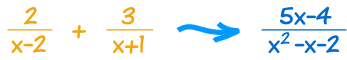2 x−2 + 3 x+1 = 2(x+1) + 3(x−2) (x−2)(x + 1)

= 2x+2 + 3x−6 x2+x−2x−2

= 5x−4 x2−x−2

（去看使用有理式来了解更多。）

……但倒转过来怎样做？（"部分分式"）。

## 部分分式分解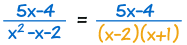一、把下面化为因式。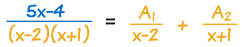二、每个因式写成一个部分分式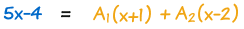三、 全部乘以下面的式，之后式子不再是分数了 四、现在来找常数！ 代入下面的式子的根（"零点"）可能会有用：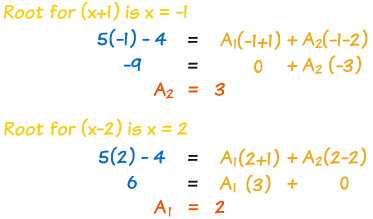得到答案了：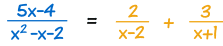……其实它可以非常困难

## 真有理式

• ：上面的次数是小于下面的次数。  真：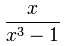上面的次数是 1 下面的次数是 3
• ：上面的次数是大于或等于下面的次数。  假：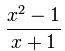上面的次数是 2 下面的次数是 1

## 把下面化为因式

### 例子：(x2-4)(x2+4)

• x2-4 可以分解为 (x-2)(x+2)
• x2+4 只可能分解为复数，所以不要把它分解

(x-2)(x+2)(x2+4)

• 线性因式，和
• 不可约二次因式

B1x + C1（你的二项式）

## 有指数的因式

### 例子：

1(x−2)3

A1x−2 + A2(x−2)2 + A (x−2)3

### 例子：

1(x2+2x+3)2

B1x + C1x2+2x+3 + B2x + C2(x2+2x+3)2

## 复杂完整的例子

x2+15(x+3)2 (x2+3)

• 因为 (x+3)2 的指数是 2，它需要两项（A1A2）。
• 同时，(x2+3) 是个二次式，所以也需要 Bx + C

x2+15(x+3)2(x2+3)  =  A1x+3 + A2(x+3)2 + Bx + Cx2+3

x2+15 = (x+3)(x2+3)A1 + (x2+3)A2 + (x+3)2(Bx + C)

x = -3 有个根（因为 x+3=0），所以用它试试：

(-3)2+15 = 0 + ((-3)2+3)A2 + 0

24 = 12A2

2 代入 A2

x2+15 = (x+3)(x2+3)A1 + 2x2+6 + (x+3)2(Bx + C)

x2+15 = (x3+3x+3x2+9)A1 + 2x2+6 + (x3+6x2+9x)B + (x2+6x+9)C

x2+15 = x3(A1+B)+x2(3A1+6B+C+2)+x(3A1+9B+6C)+(9A1+6+9C)

 x3: 0 = A1+B x2: 1 = 3A1+6B+C+2 x: 0 = 3A1+9B+6C 常数： 15 = 9A1+6+9C

 0 = A1 + B -1 = 3A1 + 6B + C 0 = 3A1 + 9B + 6C 1 = A1 + C

 0 = A1 + B -2 = 2A1 + 6B 0 = 3A1 + 9B + 6C 1 = A1 + C

 0 = A1 + B -2 = 4B 0 = 3A1 + 9B + 6C 1 = A1 + C

 A1=1/2 A2=2 B=−(1/2) C=1/2

x2+15 (x+3)2(x2+3)   =   1 2(x+3) + 2 (x+3)2 + −x + 1 2(x2+3)

（附注：我花了差不多

## 总结

• 有理式来开始（若不是真有理式，先做多项式长除）
• 因式分解下面的式子：
• 线性因式
• 或/和"不可约"二次因式
• 每个因式（和每个因式的次方）写成一个部分分式
• 全部乘以下面的式子
• 用以下方法来解系数：
• 代入下面的式子的根
• 把每个幂（x 的次方）的系数写成一个方程，形成一个线性方程组，然后解它
• 答案就出来了！